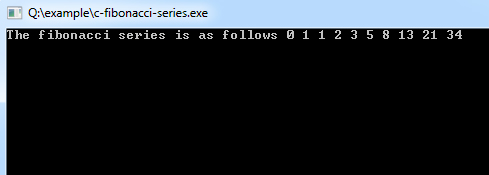# C Program to Generate the Fibonacci Series

In the Fibonacci Series, a number of the series is obtained by adding the last two numbers of the series.

Example:

Let f(n) be the n'th term.

f(0)=0;

f(1)=1;

f(n)=f(n-1)+f(n-2); (for n>=2)

Series is as follows

011

(1+0)

2 (1+1)

3 (1+2)

5 (2+3)

8 (3+5)

13 (5+8)

21 (8+13)

34 (13+21)

...and so on

### Program to Generate Fibonacci Series

Program:
``````#include<stdio.h>
int main()
{
//array fib stores numbers of fibonacci series
int i, fib;

//initialized first element to 0
fib = 0;

//initialized second element to 1
fib = 1;

//loop to generate ten elements
for (i = 2; i < 10; i++) {
//i'th element of series is equal to the sum of i-1'th element and i-2'th element.
fib[i] = fib[i - 1] + fib[i - 2];
}
printf("The fibonacci series is as follows ");
//print all numbers in the series
for (i = 0; i < 10; i++) {
printf("%d ", fib[i]);
}
return 0;
}``````
Output of program:Explanation:

The first two elements are respectively started from 0 1, and the other numbers in the series are generated by adding the last two numbers of the series using looping. These numbers are stored in an array and printed as output.